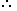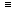# Tips and Tricks to solve problems on Calendar1. Odd Days: We are supposed to find the day of the week on a given date.For this, we use the concept of ‘odd days’.

In a given period, the number of days more than the complete weeks are called odd days.

2. Leap Year:
(i). Every year divisible by 4 is a leap year, if it is not a century.(ii). Every 4th century is a leap year and no other century is a leap year.

Note: A leap year has 366 days.

Examples:

1. Each of the years 1948, 2004, 1676 etc. is a leap year.
2. Each of the years 400, 800, 1200, 1600, 2000 etc. is a leap year.
3. None of the years 2001, 2002, 2003, 2005, 1800, 2100 is a leap year.
3. Ordinary Year: The year which is not a leap year is called an ordinary years. An ordinary year has 365 days.
4. Counting of Odd Days:
1. 1 ordinary year = 365 days = (52 weeks + 1 day.)1 ordinary year has 1 odd day.
2. 1 leap year = 366 days = (52 weeks + 2 days)1 leap year has 2 odd days.
3. 100 years = 76 ordinary years + 24 leap years= (76 x 1 + 24 x 2) odd days = 124 odd days.= (17 weeks + days)5 odd days.Number of odd days in 100 years = 5.

Number of odd days in 200 years = (5 x 2)3 odd days.

Number of odd days in 300 years = (5 x 3)1 odd day.

Number of odd days in 400 years = (5 x 4 + 1)0 odd day.

Similarly, each one of 800 years, 1200 years, 1600 years, 2000 years etc. has 0 odd days.

5. Day of the Week Related to Odd Days:
 No. of days: 0 1 2 3 4 5 6 Day: Sun. Mon. Tues. Wed. Thurs. Fri. Sat.
It was Sunday on Jan 1, 2006. What was the day of the week Jan 1, 2010?
 A. Sunday B. Saturday C. Friday D. Wednesday

Explanation:

On 31st December, 2005 it was Saturday.

Number of odd days from the year 2006 to the year 2009 = (1 + 1 + 2 + 1) = 5 days.On 31st December 2009, it was Thursday.

Thus, on 1st Jan, 2010 it is Friday.

What was the day of the week on 28th May, 2006?
 A. Thursday B. Friday C. Saturday D. Sunday

Explanation:

28 May, 2006 = (2005 years + Period from 1.1.2006 to 28.5.2006)

Odd days in 1600 years = 0

Odd days in 400 years = 0

5 years = (4 ordinary years + 1 leap year) = (4 x 1 + 1 x 2)6 odd days

```Jan.  Feb.   March    April    May
(31 +  28  +  31   +   30   +   28 ) = 148 days
```148 days = (21 weeks + 1 day)1 odd day.

Total number of odd days = (0 + 0 + 6 + 1) = 70 odd day.

Given day is Sunday.

#### What was the day of the week on 17th June, 1998?

Correct! Wrong!

#### What will be the day of the week 15th August, 2010?

Correct! Wrong!

#### Today is Monday. After 61 days, it will be:

Correct! Wrong!

#### If 6th March, 2005 is Monday, what was the day of the week on 6th March, 2004?

Correct! Wrong!

#### The last day of a century cannot be

Correct! Wrong!

QUIZ ON CALENDAR
GREAT JOB!!!!!